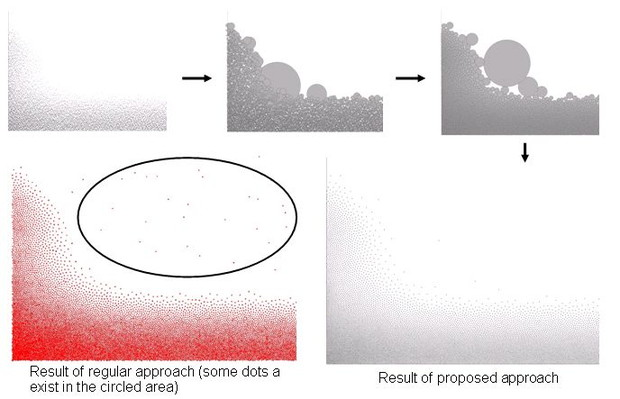## Optimization and Algorithms

IBM Research - Tokyo has a long history of research on optimization and algorithms. We have solved large and complex problems in the real world by applying various techniques of optimization. Representative cases that we have worked on since 1990 include optimization systems for the iron and steel industry and for logistics. Simple applications of standard approaches to these problems fail because of their complexity, which comes from their many constraints and large combinatorial structures. Effective solutions require precise understanding of the clients' problems, models for as tractable optimization problems, and efficient algorithms that exploit the particular structures of the system. Meanwhile, the performance of such optimization solvers as IBM's ILOG CPLEX (R) have greatly improved, and it is becoming essential to exploit these existing tools effectively within the overall solutions, particularly for the faster development of complete solutions.

Recently we have also been studying the optimization of stochastic systems, where the information necessary for optimization is not deterministic. Optimization based on deterministic values does not lead to improvement in the real world if the deterministic values are actually stochastic. We assume that the input information is stochastic, and model each problem so that it can be optimized with mathematical programming or Markov decision processes. Specific applications include optimizations for allocating computational resources to virtual machines and optimizations for controlling power generation and battery use. The resource consumption of the virtual machines and the electric power consumption are not known in advance, but our optimizations work with predicted values as stochastic input. As advanced fundamental research, we are also studying risk-sensitive optimization (in T. Osogami, "Iterated risk measures for risk-sensitive Markov decision processes with discounted cost," UAI 2011). In particular, we are investigating the risk metrics for representing the preferences that cannot be represented by expectations or expected utility functions. We are also developing algorithms for efficient optimizations with respect to such risk metrics.

Some of our recent contributions involve the analysis of random walks （in T. Osogami and R. Raymond, "Semidefinite optimization for transient analysis of queues," ACM SIGMETRICS 2010）. We proposed an algorithm for analyzing properties of random walks, making full use of optimization theories and techiques such as semidefinite optimization, duality theory, and optimality conditions. Random walks are one of the fundamental stochastic processes, and their analysis has many applications, including the analysis of queues, the analysis of algorithms, and the design of experiments. We also believe that the new analytical techniques for random walks will find applications in the analysis of more complex stochastic processes. We have shown that our approach finds upper and lower bounds on the probability that a random walk crosses a given region with an accuracy that is higher than those possible with existing approaches including large deviations and martingales. Also, our approach can give the bounds in closed forms, which allowed us to discover properties of queues in transient periods, which were little understood until recently（in T. Osogami and R. Raymond, "Simple bounds for a transient queue," IEEE/IFIP DSN 2011). We believe that we can extend this new proposed approach to optimizing stochastic systems and are continueing our basic research in this area.Fig.1 - analyzing properties of random walks making use of semidefinite optimization

Other representative contributions involve the classic shortest path problem. In particular, using SIMD (Single Instruction Multiple Data) instructions, we developed an efficient algorithm for finding the shortest paths for all of the pairs of nodes on a sparse graph, a problem corresponding to such real-wold scenarios as road networks (in H.Yanagaisawa, IPDPS 2010). Our approach runs an order of magnitude faster than the repeated application of the traditional Dijkstra algorithm. This is the world's first algorithm that uses SIMD instructions for accelerating the shortest path search for a sparse graph.Fig.2 - algorithm for finding shortest paths for all of the pairs of nodes on a sparse graph

We have also made major contributions in packing problems. The problem of packing two or three dimensional objects without collisions has a wide range of applications in the industry such as positioning wire harnesses. We used a generic approach that approximates the objects with a set of spheres and then optimizes the locations of the spheres. The approach of approximation with spheres has the advantages for unconstrained rotations of the objects (in T.Imamichi and H.Nagamochi, SLS2007).Fig.3 - approximating the objects with a set of balls and then finding the placement of the balls

We also successfully applied the optimization technique for packing problems with circle-based approximations to generate dot patterns for producing liquid crystal displays. These patterns are extremely smooth and produce almost no moire patterns（in T. Imamichi et al., “Nonlinear Optimization to Generate Non-overlapping Random Dot Patterns,” WSC 2011）. Regular dot patterns tend to result in highly visible moire patterns, while dot patterns based on pseudo-random numbers or low discrepancy sequences tend to be visibly rough. Ten years ago, we first successfully generated dot patterns of extremely high quality by combining low discrepancy sequences and a model of molecular dynamics. The new optimization technique for packing problems can be used to more quickly generate dot patterns whose quality is even higher than that with the prior approach.Fig.4 - example of generating dot patterns of super high quality